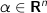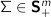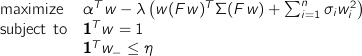# CVXGEN: Code Generation for Convex Optimization

## Example: Portfolio optimization

This example, from finance, is a basic portfolio optimization problem. For some more details, see Boyd and Vandenberghe,4.6.3.

## Optimization problem

We are given the parameters

•(mean returns)

•(risk aversion parameter)

•(factor exposure matrix)

•(factor covariance matrix)

•(idiosyncratic or asset-specific variance)

•(leverage limit)

and wish to choose asset weights

•to minimize our risk-adjusted mean return, subject to a budget constraint and a limit on our total short position.

Thus, we have the optimization problem## CVXGEN code

dimensions
n = 50  # assets.
m = 10  # factors.
end

parameters
alpha (n)  # vector of mean returns.
lambda positive  # risk aversion.
sigma (n) positive  # idiosyncratic (asset-specific) variance.
eta positive  # limit on total short position.
F (m,n)  # factor exposure matrix.
Sigma (m,m) psd  # factor covariance matrix.
end

variables
w (n)  # asset weights.
end

maximize